## 2.7Conservation of momentum

The law of conservation of momentum can be written4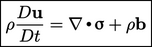(2.19)
where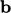represents any body force per unit mass. Body forces represent any force which does not act at a bounding surface, including those that act at a distance, such as gravitational force.

The equation is derived by considering the time rate of change of the momentum of a mass of particles. We consider a volume of material of ﬁxed mass moving through space and therefore present rate of change by the material derivative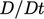.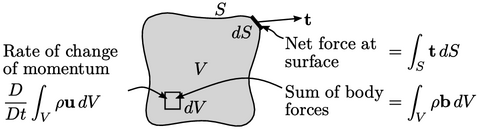Applying Gauss’s theorem and Eq. (2.16 ), the surface force is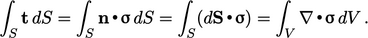(2.20)
Equating the rate of change of momentum to the forces and, noting mass is ﬁxed so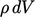is constant in time, gives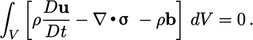(2.21)
The integrand must equal 0, resulting in Eq. (2.19).

Divergence was described in section 2.4 as the ﬂux across a surface per unit volume as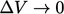. The divergence of stress similarly represents the stress ﬂux across the surface, i.e. force, per unit volume as, given by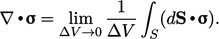(2.22)
Eq. (2.19 ) speciﬁcally relates to linear momentum. Instead, conservation of angular momentum5, in the absence of any “couple stresses” that generate a moment ﬁeld, is given by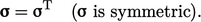(2.23)
The derivation of Eq. (2.23 ) is fairly complex so is omitted here.

### Tensor symmetry

Symmetry in a tensor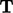refers to components being symmetric about the diagonal, i.e.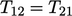,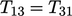and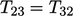. The transpose of a tensor, denoted by the ‘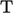’ superscript, switches components across the diagonal such that: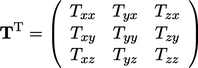(2.24)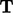is therefore symmetric if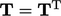. A skew (anti-symmetric) tensor has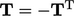. A tensor can be decomposed into symmetric and skew parts by: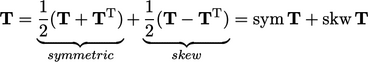(2.25)

4Cauchy’s ﬁrst law of motion (1827)
5Cauchy’s second law of motion (1827)

Notes on CFD: General Principles - 2.7 Conservation of momentum